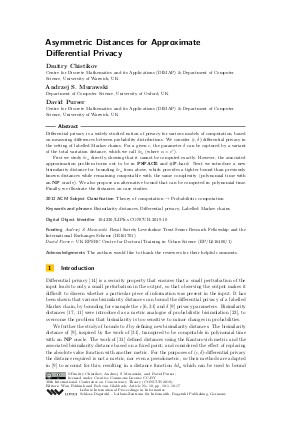Document# Asymmetric Distances for Approximate Differential Privacy

### Authors Dmitry Chistikov, Andrzej S. Murawski, David Purser## File

LIPIcs.CONCUR.2019.10.pdf
• Filesize: 0.67 MB
• 17 pages

## Acknowledgements

The authors would like to thank the reviewers for their helpful comments.

## Cite As

Dmitry Chistikov, Andrzej S. Murawski, and David Purser. Asymmetric Distances for Approximate Differential Privacy. In 30th International Conference on Concurrency Theory (CONCUR 2019). Leibniz International Proceedings in Informatics (LIPIcs), Volume 140, pp. 10:1-10:17, Schloss Dagstuhl - Leibniz-Zentrum für Informatik (2019)
https://doi.org/10.4230/LIPIcs.CONCUR.2019.10

## Abstract

Differential privacy is a widely studied notion of privacy for various models of computation, based on measuring differences between probability distributions. We consider (epsilon,delta)-differential privacy in the setting of labelled Markov chains. For a given epsilon, the parameter delta can be captured by a variant of the total variation distance, which we call lv_{alpha} (where alpha = e^{epsilon}). First we study lv_{alpha} directly, showing that it cannot be computed exactly. However, the associated approximation problem turns out to be in PSPACE and #P-hard. Next we introduce a new bisimilarity distance for bounding lv_{alpha} from above, which provides a tighter bound than previously known distances while remaining computable with the same complexity (polynomial time with an NP oracle). We also propose an alternative bound that can be computed in polynomial time. Finally, we illustrate the distances on case studies.

## Subject Classification

##### ACM Subject Classification
• Theory of computation → Probabilistic computation
##### Keywords
• Bisimilarity distances
• Differential privacy
• Labelled Markov chains

## Metrics

• Access Statistics
• Total Accesses (updated on a weekly basis)
0